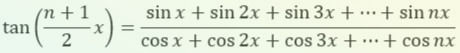top of page
Search
• vc9493

# Beautiful Sum of Sines and Cosines Formulas!

Updated: Mar 22

TheoremProof: The result is very reminiscent of the sum of an arithmetic series formula,

1+2+3+···+n = n(n+1)/2. We begin by writing out the sum forwards and in reverse, let

On each [...] using the sum-to-product formula,we get,

Factorising,

The clever step is to now multiply both sides by sin(x/2)...

In the [...] each sin(*)cos(*) term can be rewritten using the product-to-sum formula,So we get,

Now we note that sin((2x-nx)/2) = -sin((-2x+nx)/2) and sin((4x-nx)/2) = -sin((-4x+nx)/2) and so forth. This means that all terms inside [...] cancel out except the first and last (telescopic cancelling). So we get,

TheoremProof: Following the same procedure, we write

Applying the sum-to-product formula,we get,

Factorising,

The same cancelling argument as above leads to

If we now divide the two formulas, we get the elegant resultBoth formulas can also be proved by taking the real and imaginary parts of the geometric series,

From the summation formula this becomes,

Therefore,

The imaginary part of the RHS is:

Hence,

In equation * if we take the real part, we get

Hence,